# Hypergeometrical Universe

## The Kaons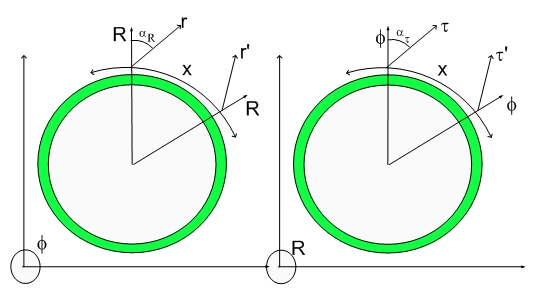The Kaons

The Kaons is a detour. Up to know we only analysed particles that contained Electron-Neutrinos (Electron-Proton-Electron transmutation transitions) or Muon-Neutrinos (Electron-Positron-Electron transmutation transitions)

The Kaons will involve the last known neutrino: The Tau Neutrino.

Let's start with the Kaon Minus assignment: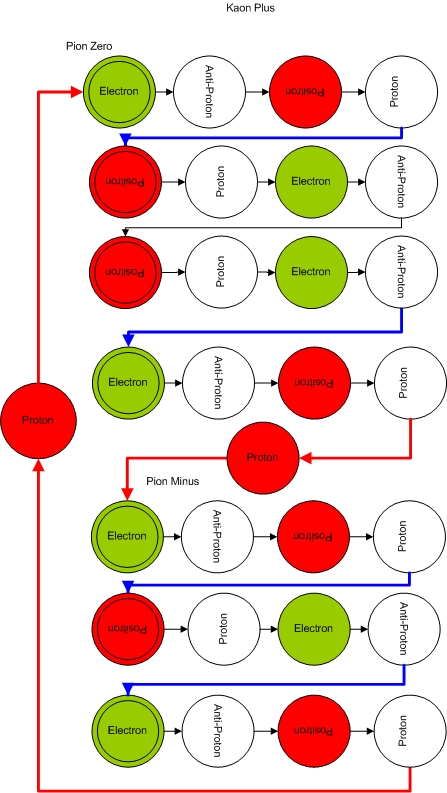which decays into a Pion Minus plus a Pion Zero.

Similarly the Kaon Plus decays into a Pion Plus and a Pion Zero.Secondary decay channels are:
KaonPlus -> MuonPlus + MuonNeutrino

KaonMinus -> MuonMinus +MuonAntiNeutrino

Below we have an irreducible representation of Kaon Minus and Plus. Notice that the number of transmutation lines is kept as well as the their respective charges.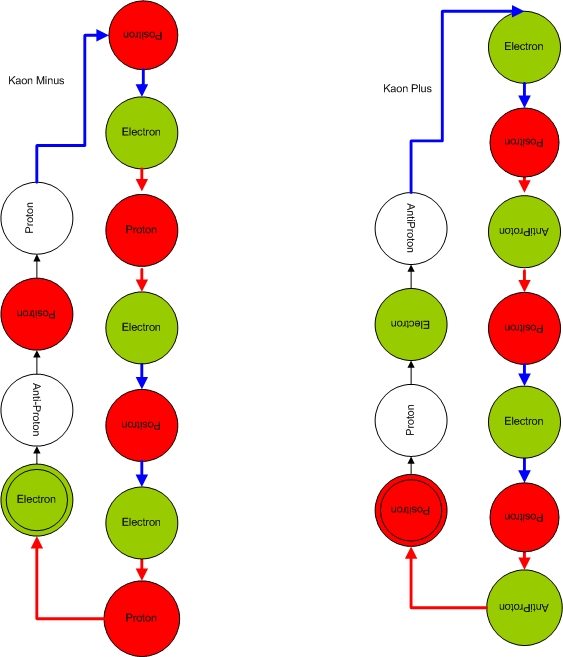This assignment goes hand in hand with the Muon assignments...:)

The KaonMinus -> MuonMinus +MuonAntiNeutrino decay is shown below: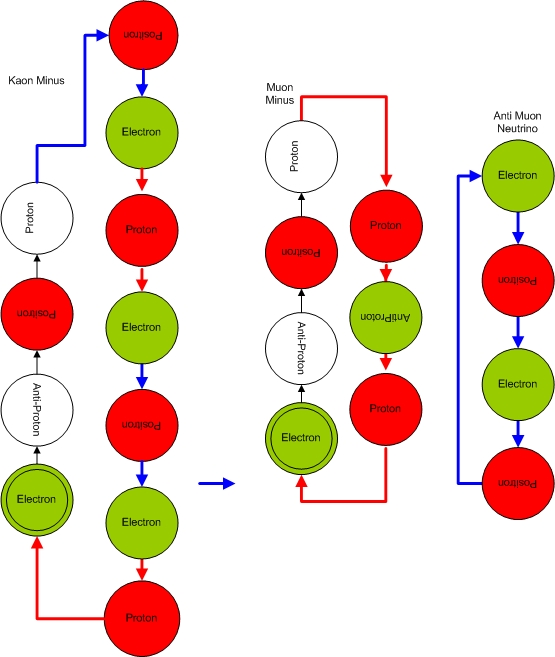The KaonPlus -> MuonPlus + MuonNeutrino decay is just changing red by green and electron by positron, and antiProton by Proton etc...:)

The Neutrino Tau is the last Neutrino to be considered and it is basically the same thing as the irreducible representqation of the Kaon. Neutrino Tau also decays into a MuonPlus plus a MuonNeutrino....:)

The last of the Kaons is the Kaon Zero shown below: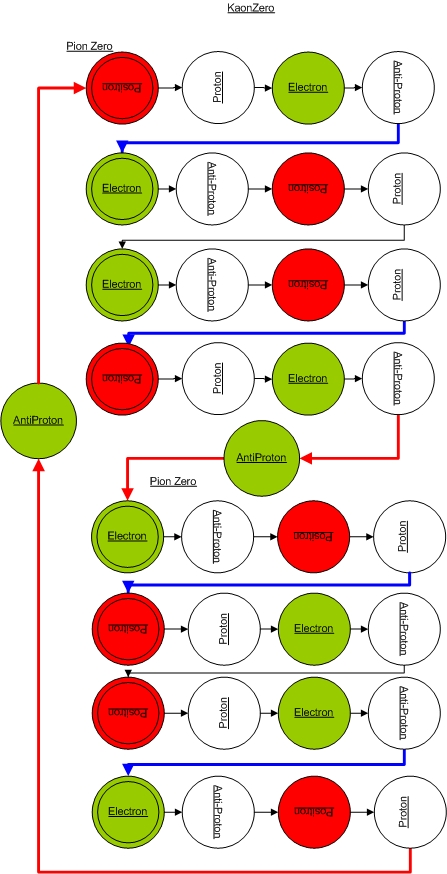The anti-KaonZero is shown below: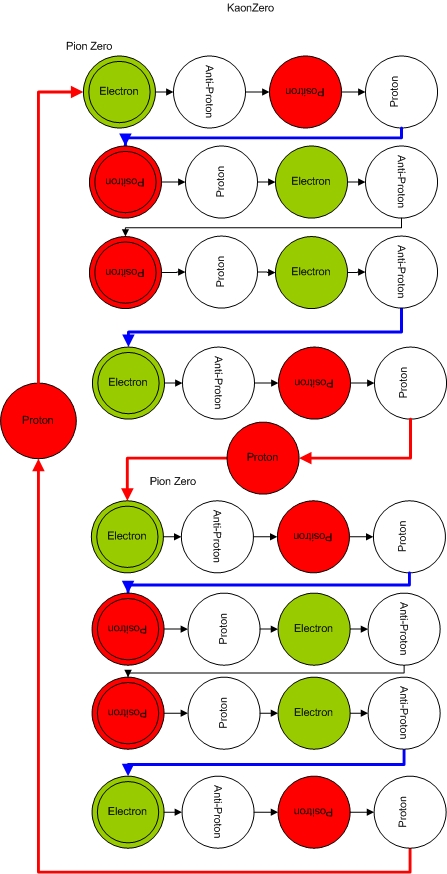And Yes, the Kaon Zero is also a Majorama coherence.

Cheers,

MP

Currently unrated

or

RSS / Atom